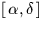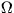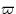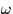Next: SLA_PM - Proper Motion
Up: SUBPROGRAM SPECIFICATIONS
Previous: SLA_PLANET - Planetary Ephemerides

## SLA_PLANTE -of Planet from Elements

ACTION:
Topocentric apparentof a Solar-System object whose heliocentric orbital elements are known.

CALL:
CALL sla_PLANTE ( DATE, ELONG, PHI, JFORM, EPOCH, ORBINC, ANODE, PERIH, AORQ, E, AORL, DM, RA, DEC, R, JSTAT)

GIVEN:

 D DATE MJD of observation (JD-2400000.5) ELONG,PHI D observer's longitude (east +ve) and latitude radians) JFORM I choice of element set (1-3, see Note 4, below) EPOCH D epoch of elements (t0 or T, TT MJD) ORBINC D inclination (i, radians) ANODE D longitude of the ascending node (, radians) PERIH D longitude or argument of perihelion (or, radians) AORQ D mean distance or perihelion distance (a or q, AU) E D eccentricity (e) AORL D mean anomaly or longitude (M or L, radians, JFORM=1,2 only) DM D daily motion (n, radians, JFORM=1 only)

RETURNED:

 D RA,DEC topocentric apparent(radians) R D distance from observer (AU) JSTAT I status: 0 = OK -1 = illegal JFORM -2 = illegal E -3 = illegal AORQ -4 = illegal DM -5 = numerical error

NOTES:
1.
DATE is the instant for which the prediction is required. It is in the TT timescale (formerly Ephemeris Time, ET) and is a Modified Julian Date (JD-2400000.5).
2.
The longitude and latitude allow correction for geocentric parallax. This is usually a small effect, but can become important for Earth-crossing asteroids. Geocentric positions can be generated by appropriate use of the routines sla_EVP and sla_PLANEL.
3.
The elements are with respect to the J2000 ecliptic and equinox.
4.
Three different element-format options are available, as follows.

JFORM=1, suitable for the major planets:


EPOCH 		 = 		 epoch of elements t0 (TT MJD)
ANODE 		 = 		 longitude of the ascending node(radians)
PERIH 		 = 		 longitude of perihelion(radians)
AORQ 		 = 		 mean distance a (AU)
E 		 = 		 eccentricity e
AORL 		 = 		 mean longitude L (radians)
DM 		 = 		 daily motion n (radians)


JFORM=2, suitable for minor planets:


EPOCH 		 = 		 epoch of elements t0 (TT MJD)
ANODE 		 = 		 longitude of the ascending node(radians)
PERIH 		 = 		 argument of perihelion(radians)
AORQ 		 = 		 mean distance a (AU)
E 		 = 		 eccentricity e
AORL 		 = 		 mean anomaly M (radians)


JFORM=3, suitable for comets:


EPOCH 		 = 		 epoch of perihelion T (TT MJD)
ANODE 		 = 		 longitude of the ascending node(radians)
PERIH 		 = 		 argument of perihelion(radians)
AORQ 		 = 		 perihelion distance q (AU)
E 		 = 		 eccentricity e

5.
Unused elements (DM for JFORM=2, AORL and DM for JFORM=3) are not accessed.Next: SLA_PM - Proper Motion
Up: SUBPROGRAM SPECIFICATIONS
Previous: SLA_PLANET - Planetary Ephemerides

SLALIB --- Positional Astronomy Library